# One Step Equations Lesson Plan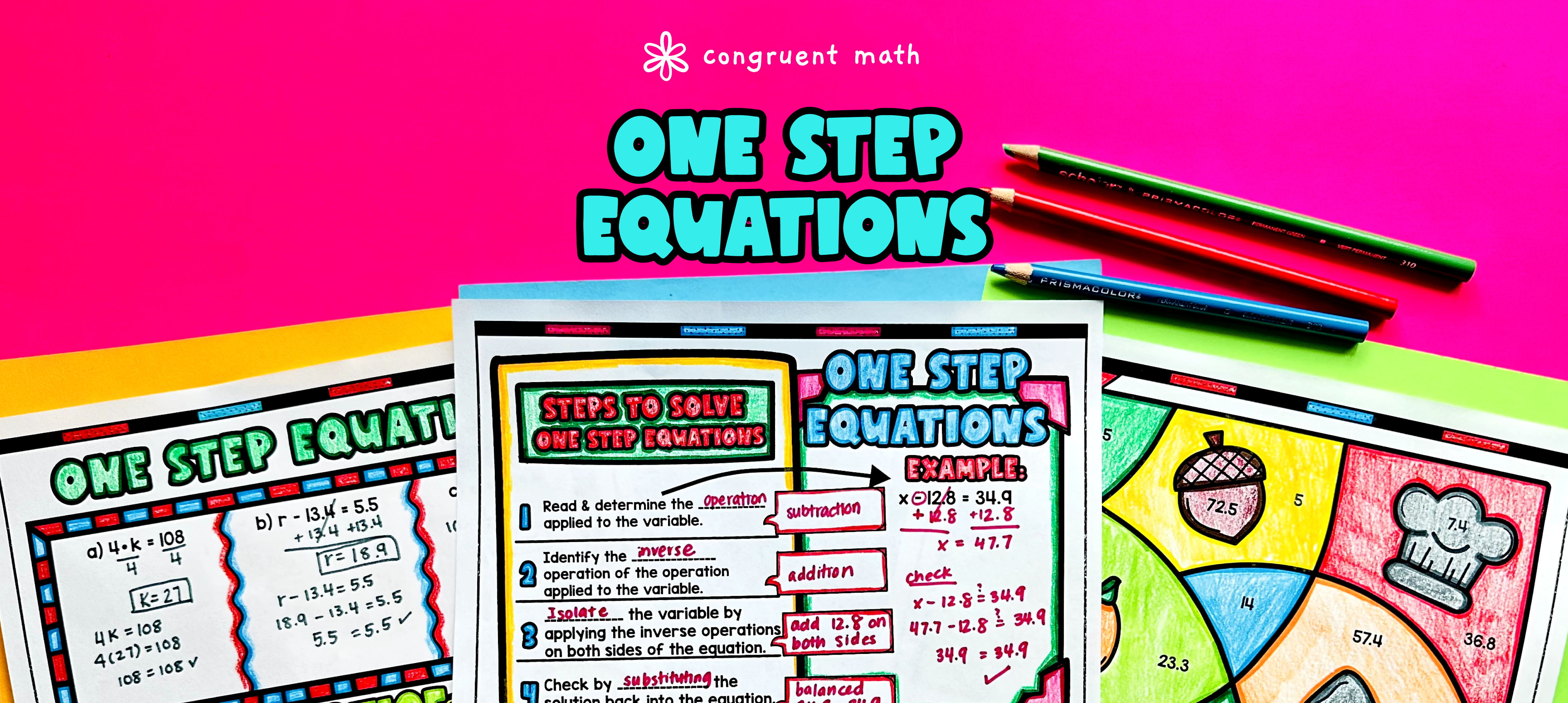Pin This

## Overview

Ever wondered how to teach one-step equations with positive solutions in an engaging way to your 6th-grade students?

In this lesson plan, students will learn about solving one-step equations and their real-life applications. Through artistic, interactive guided notes, checks for understanding, a doodle and color by number activity, and a maze worksheet, students will gain a comprehensive understanding of one-step equations.

The lesson culminates with a real-life example that explores how unit conversions can be applied in everyday situations. This lesson is not only effective for introductory lessons but also for reteaching, spiral review, homework, sub plans, and quiz, test, and exam preparation. By the end of the lesson, students will be able to confidently solve one-step equations with positive solutions.

## Get the Lesson Materials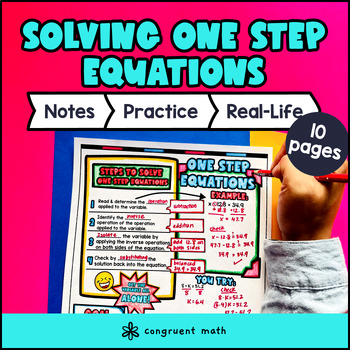\$4.25

## Learning Objectives

After this lesson, students will be able to:

• Solve one-step equations with positive solutions

• Use inverse operations to isolate the variable in one-step equations

• Check their solutions to one-step equations

• Explain the real life applications of one step equations

## Prerequisites

Before this lesson, students should be familiar with:

• Basic operations (addition, subtraction, multiplication, and division) of whole numbers and decimals

## Key Vocabulary

• One-step equations

• Inverse operations

## Procedure

### Introduction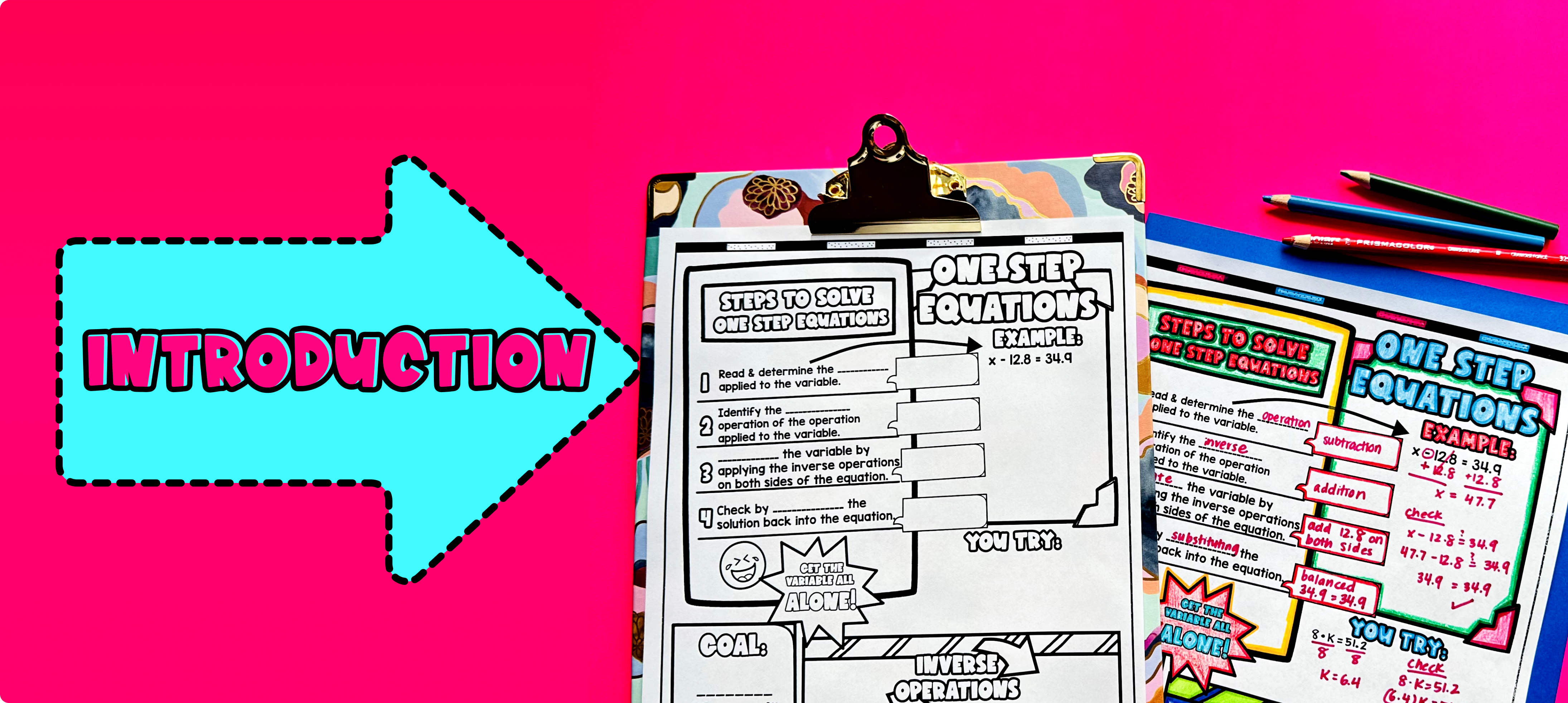Pin This

As a hook, ask students why it is important to be able to solve one-step equations in real-life situations. Refer to the last page of the guided notes as well as the FAQs below for ideas.

Use the first page of the guided notes to introduce the steps to solve one-step equations. Walk through the process of isolating the variable using inverse operations. Emphasize the importance of performing the same operation on both sides of the equation to maintain equality.

Based on student responses, reteach any concepts that students need extra help with. If your class has a wide range of proficiency levels, you can pull out students for reteaching, and have more advanced students begin work on the practice exercises.

### Practice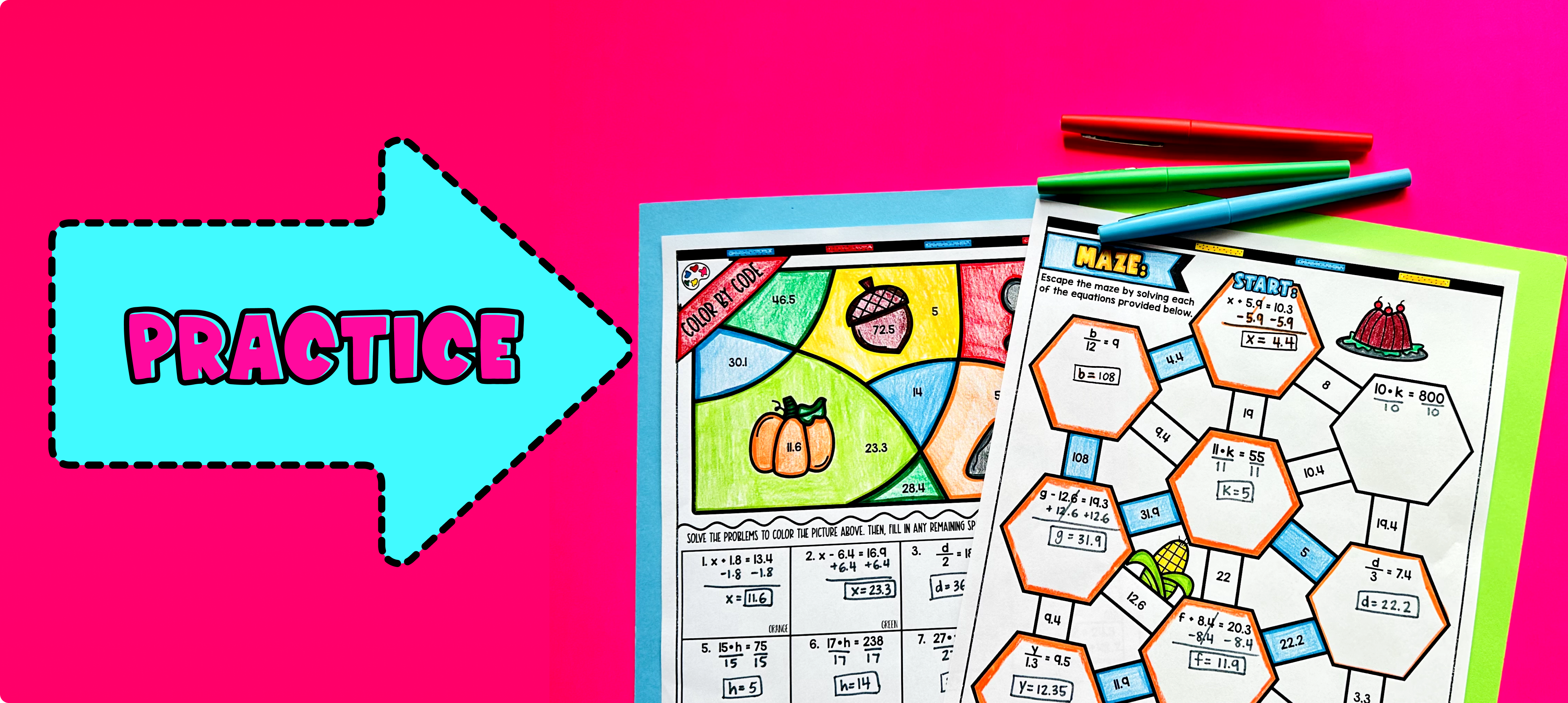Pin This

Have students practice solving one-step equations with positive solutions using the practice worksheet (page 2 of guided notes) included in the resource. The practice worksheets include color by code, maze, and problem sets for students to work on.

Walk around the classroom to answer any student questions and provide assistance as needed.

For fast finishers, they can further reinforce their skills by working on fun worksheets including color by number (page 3 of guided notes) and a maze activity (page 4 of guided notes). You can assign this activity as homework for the remainder of the class, allowing students to continue practicing and applying their knowledge.

### Real-Life Application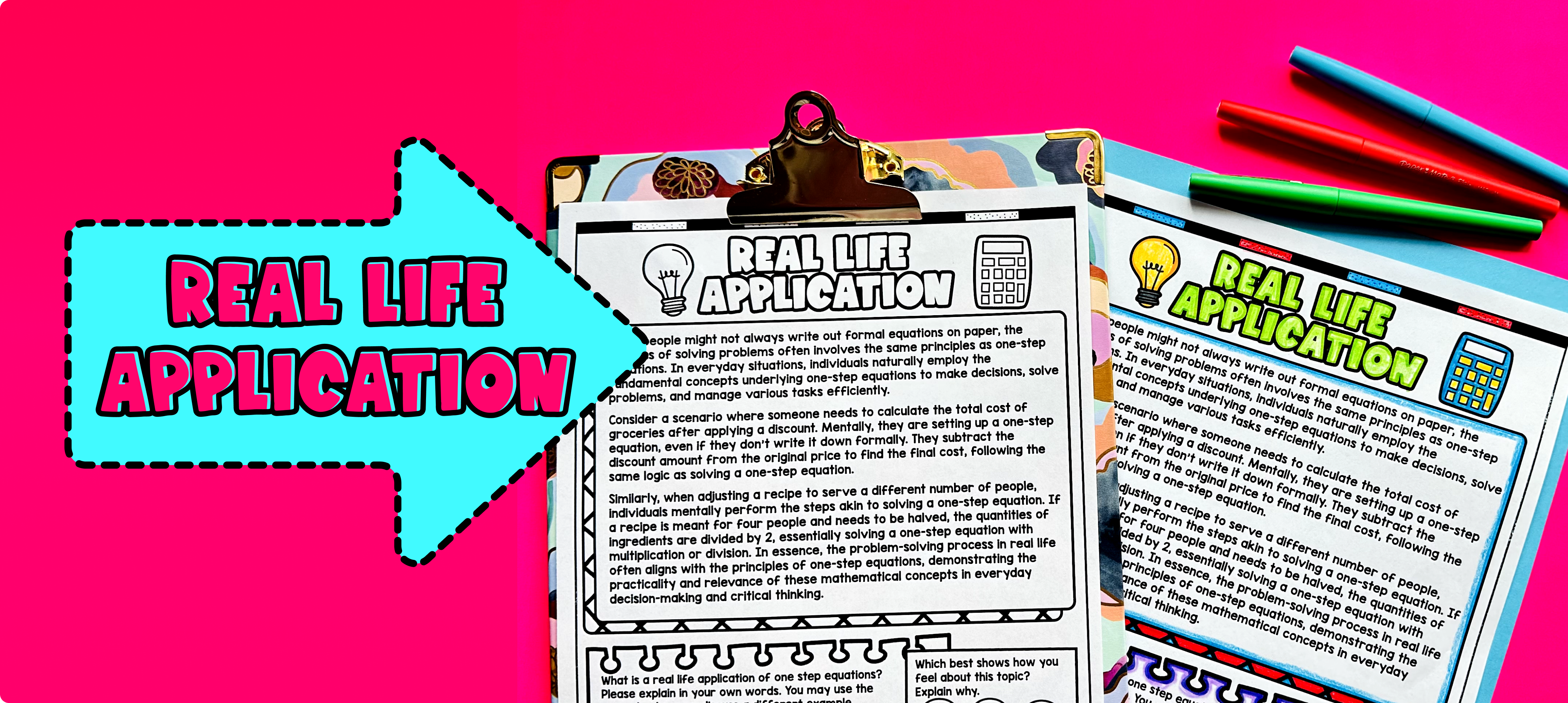Pin This

Use the last page of the guided notes to bring the class back together, and introduce the concept of real-life applications of solving one-step equations with positive solutions. Explain to students that one-step equations can be used to solve problems in various real-life situations. For example, they can be used to solve problems related to buying items, calculating distances, or determining cooking measurements.

Encourage students to think about how they can use one-step equations in their daily lives. Ask them to share any real-life situations they can come up with where solving one-step equations with positive solutions would be helpful. This can be done through a class discussion or in small groups.

Refer to the FAQ section of the resource for additional ideas on how to teach real-life applications of solving one-step equations with positive solutions.

## Extensions

If you’re looking for digital practice for solving one-step equations with positive solutions, try my Pixel Art activities in Google Sheets. Every answer is automatically checked, and correct answers unlock parts of a mystery picture. It’s incredibly fun, and a powerful tool for differentiation.

Here’s 2 activities to explore: